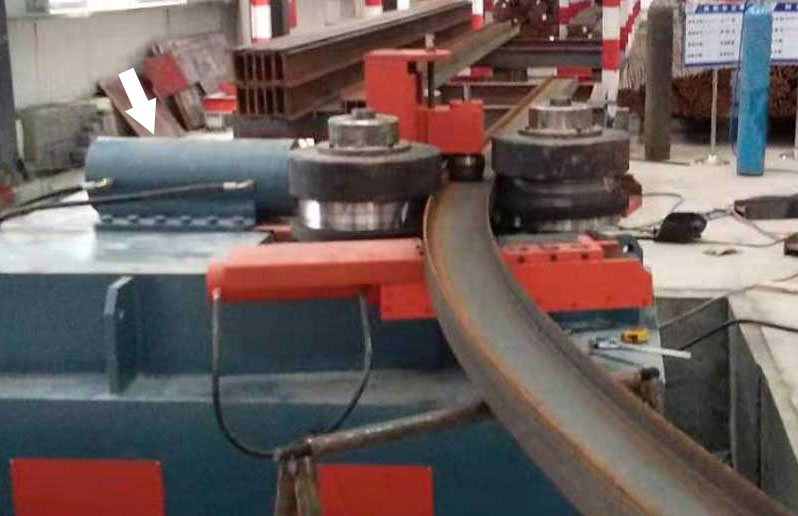# Unveiling the Secrets of Bending Stiffness in BeamsIn the world of structural engineering and mechanics, the bending stiffness of a beam stands as a fundamental concept that engineers and designers grapple with regularly. Whether in the construction of a skyscraper’s support beams or the creation of a flexible bridge, understanding the behavior of beams under bending loads is essential.

## What is Bending Stiffness?

Bending stiffness, denoted as “EI,” is a critical mechanical property that characterizes a beam’s resistance to bending deformation when subjected to loads. It is a measure of how rigid or flexible a beam is and plays a pivotal role in determining its structural behavior.

## The Significance of Bending Stiffness

Understanding the importance of bending stiffness in beams is crucial for several reasons:

1. Structural Integrity: Bending stiffness is a primary factor in ensuring that a beam can support its intended load without excessive deflection or failure.
2. Design Optimization: Engineers rely on bending stiffness to design beams that meet specific performance criteria, balancing factors like strength, deflection, and material usage.
3. Load Analysis: Bending stiffness is essential for predicting how a beam will behave under various loads, guiding decisions regarding safety and performance.
4. Material Selection: It aids in selecting the right materials and dimensions to achieve the desired stiffness characteristics for a given application.

## Calculating Bending Stiffness

Calculating bending stiffness (EI) involves a systematic approach that considers both material properties and geometric factors. Here’s a step-by-step guide:

### Determine Material Properties

Identify the material properties required for the calculation, including the modulus of elasticity (E) and the second moment of area (I) for the cross-sectional shape. E describes the material’s stiffness, while I characterizes the shape’s resistance to bending.

### Define the Cross-Section

Precisely define the cross-sectional shape of the beam. This involves measuring dimensions, such as the width (b) and height (h), as well as any variations in thickness or shape.

List of Cross-Sectional Dimensions for Bending Stiffness Calculation:

• Width (b)
• Height (h)
• Thickness (t)

### Calculate the Second Moment of Area (I)

Depending on the shape of the cross-section, use the appropriate formula to calculate the second moment of area. Common shapes like rectangles, circles, and I-beams have well-established formulas.

Compute Bending Stiffness (EI)

Utilize the formula for bending stiffness: S=(E*I)/L​, where E is the modulus of elasticity, and I is the second moment of area.

List of Symbols for Bending Stiffness Calculation

• EI: Bending Stiffness
• E: Modulus of Elasticity
• I: Second Moment of Area
• Plug in the values for E and I into the formula to calculate bending stiffness.

### Interpret the Results

The calculated bending stiffness (EI) represents the beam’s resistance to bending deformation. A higher EI value signifies greater stiffness, while a lower value indicates greater flexibility.List of Factors Influencing Bending Stiffness:

• Material properties (E)
• Geometric dimensions (I)
• Cross-sectional shape

## Conclusion

Bending stiffness is a foundational concept in structural engineering and mechanics, shaping the design and performance of beams in various applications. By comprehending the step-by-step process of calculating bending stiffness, engineers and designers can make informed choices about materials, cross-sectional shapes, and structural dimensions. This knowledge empowers them to create beams that meet specific requirements, ensuring safety, efficiency, and durability in a wide range of structural projects.